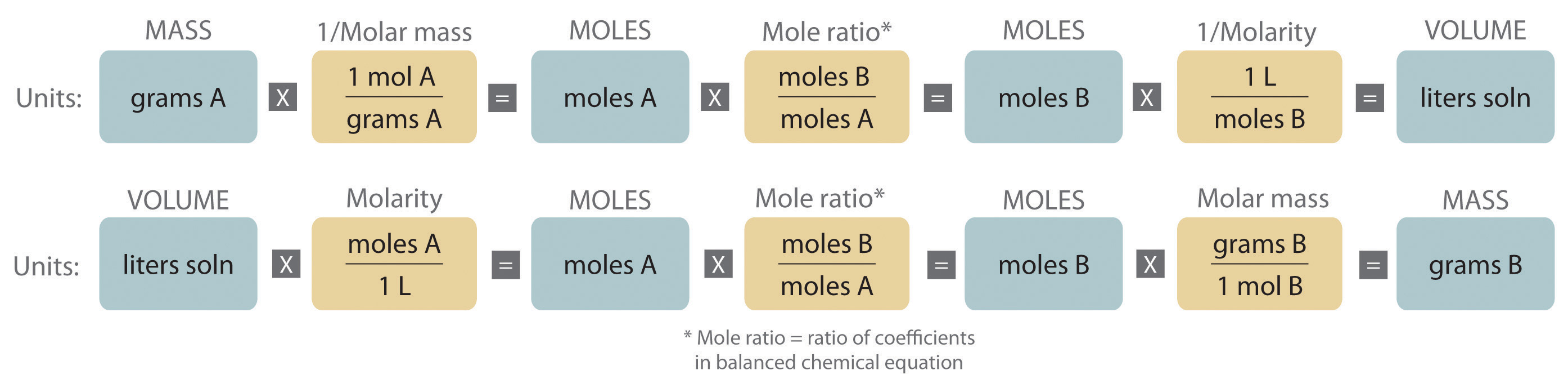How can I convert percent concentration to molarity?

Aug 31, 2014

Here's one way to do it.

Explanation:

Example

Concentrated hydrochloric acid is usually available at a concentration of 37.7% by mass. The density of the solution is 1.19 g/mL. What is its molar concentration?

Solution

Step 1. Calculate the mass of 1 L of solution.

"Mass of solution" = 1000 color(red)(cancel(color(black)("mL soln"))) × "1.19 g soln" /(1color(red)(cancel(color(black)("mL soln")))) = "1190 g soln" (3 significant figures + 1 guard digit)

Step 2. Calculate the mass of $\text{HCl}$.

$\text{Mass of HCl" = 1190 color(red)(cancel(color(black)("g soln"))) × (37.7"g HCl")/(100color(red)(cancel(color(black)("g soln")))) = "448.6 g HCl}$

Step 3. Calculate the moles of $\text{HCl}$.

448.6 color(red)(cancel(color(black)("g HCl"))) × "1 mol HCl"/(36.46color(red)(cancel(color(black)("g HCl")))) = "12.30 mol HCl"

Step 4. Calculate the molarity of the HCl.

$\text{molarity" = "moles of HCl"/"litres of solution" = "12.30 mol"/"1 L" = "12.3 mol/L}$

Here's a summary of the types of calculations we were using aboveAnd here's a video about converting mass % to molarity.